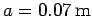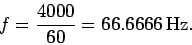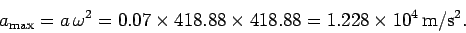Next: Worked example 11.2: Block Up: Oscillatory motion Previous: Uniform circular motion

## Worked example 11.1: Piston in steam engine

Question: A piston in a stream engine executes simple harmonic motion. Given that the maximum displacement of the piston from its centre-line is, and that the mass of the piston is, find the maximum velocity of the piston when the steam engine is running at 4000rev./min. What is the maximum acceleration?

Answer: We are told that the amplitude of the oscillation is. Moreover, when converted to cycles per second (i.e., hertz), the frequency of the oscillation becomesHence, the angular frequency isConsulting Tab. 4, we note that the maximum velocity of an object executing simple harmonic motion is. Hence, the maximum velocity isLikewise, according to Tab. 4, the maximum acceleration is given byNext: Worked example 11.2: Block Up: Oscillatory motion Previous: Uniform circular motion
Richard Fitzpatrick 2006-02-02